Security Market Indexes | IFT World
IFT Notes for Level I CFA® Program
IFT Notes for Level I CFA® Program

# Part 1

## 1.  Introduction

An index is an indicator, sign, or measure of something. Since an index is a single measure and reflects the performance of the entire security market, it makes it easy for investors to measure and track performance.

Security market indexes were first introduced as a simple measure to reflect the performance of the U.S. stock market. Dow Jones Average, the world’s first security market index, was introduced in 1884 comprising only nine railroad and two industrial companies. Until then, investors gathered data of individual securities to assess performance.

Now, security market indexes have multiple uses that help an investor track performance of various markets, estimate risk, and evaluate the performance of an investment. Major indexes include S&P 500, FTSE, and Nikkei.

This reading defines what a security market index is, explains how to calculate the returns of an index, how indexes are constructed, the need for market indexes, and the types of indexes.

## 2.  Index Definition and Calculations of Value and Returns

A security market index measures the value of different target markets such as security markets, market segments, and asset classes. The index value is calculated on a regular basis using actual or estimated prices of constituent securities. Constituent securities are the individual securities comprising an index.

Each index often has two versions based on how the return is calculated:

• A price return index or price index measures only the percentage change in price of the constituent securities within the index.
• A total return index considers the prices of constituent securities and the reinvestment of all income (dividend and/or interest) since inception.

The value of both versions will be the same at inception. However, as time passes, the value of the total return index will exceed the value of the price return index.

### 2.1. Calculation of Single-Period Returns

The price return and total returns for an index can be computed using the following formulae:

Price return of an index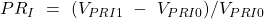where

PR1= price return of an index (in decimal)

VPRI1= value of the price return index at the end of the period

VPRI0= value of the price return index at the beginning of the period

Total return of an index: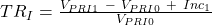where

TR1 = total return of the index portfolio

VPRI1= value of the price return index at the end of the period

VPRI0= value of the price return index at the beginning of the period

Inc1= income from all the securities in the index over the period

### 2.2. Calculation of Index Values over Multiple Time Periods

Once returns are calculated for each period, the calculation of index values over multiple periods is done by geometrically linking returns.

For example, if the value of a total return index at the start of period 1 is 100 and the total returns over three periods are: 16%, 11%, and -4%, the index value at the end of period three will be: 100 x 1.16 x 1.11 x 0.96 = 123.61.

## 3.  Index Construction

Constructing and managing an index is similar to building a portfolio of securities. The difference is that an index is a paper portfolio but a real portfolio consists of actual securities. The following factors must be considered when constructing a security index:

• Target market. E.g., U.S. equities.
• Security selection. E.g., large cap securities.
• Weight allocated to each security in the index.
• Index rebalancing.

### 3.1.     Target Market and Security Selection

The target market determines the investment universe. It can be defined broadly (for example, all U.S. equities) or narrowly (for example, large cap telecom stocks in China). If the target market is U.S. equities, then the constituent securities for the index will come from the universe of U.S. equities. The target market may also be based on market capitalization, asset class, geographic region, industries, sizes, exchange, and/or other characteristics.

### 3.2.     Index Weighting

Index weighting determines how much of each security to include in the index. This decision impacts index value. We will see four methods to determine the weight of the securities in an index:

• Price weighting
• Equal weighting
• Market-capitalization weighting
• Fundamental weighting

For each weighting method, there could be a price return index or a total return index.

Price Weighted Index

The weight of each security is calculated by dividing its price by the sum of all prices. One example of a price-weighted index is the Dow Jones Industrial Average.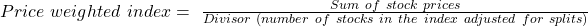Example

Consider three securities A, B and C comprising an index with the following beginning of period (BOP), and end of period (EOP) values. Using a divisor of 3, compute a) the index value, b) the price return and the total return.

 Beginning of period price Beginning of period weight End of period price Dividends/share A 4 20% 2 0 B 6 30% 6 1 C 10 50% 14 2

Solution:

Using the above equation, value of the index at start of the period =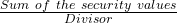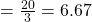Value of index at end of the period =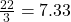Price return =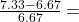9.89%
Dividend return =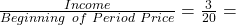15%

Total return = Price return + Dividend return ≈ 25%

To remove the impact of stock splits, security addition or deletion; the divisor is adjusted.

Example

In the previous example, if there is a 2-for-1 split in stock C during the period, what is the impact on index value and return calculations?

Solution:

Initial divisor was 3 and end of index value = 7.33. End of period price of C is 7 after the split.

The divisor must be adjusted to prevent the stock split and the new weights from changing the value of the index.

Value of index =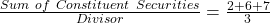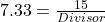Divisor = 2.05

Note that every time there is a stock split, the value of the divisor will decrease.

Advantage of price weighted index: Simplicity.

Limitations of price weighted index:

• Results in arbitrary weights for securities.
• If the price of a security is high, it will receive a relatively high weight, even though its market capitalization might be low.